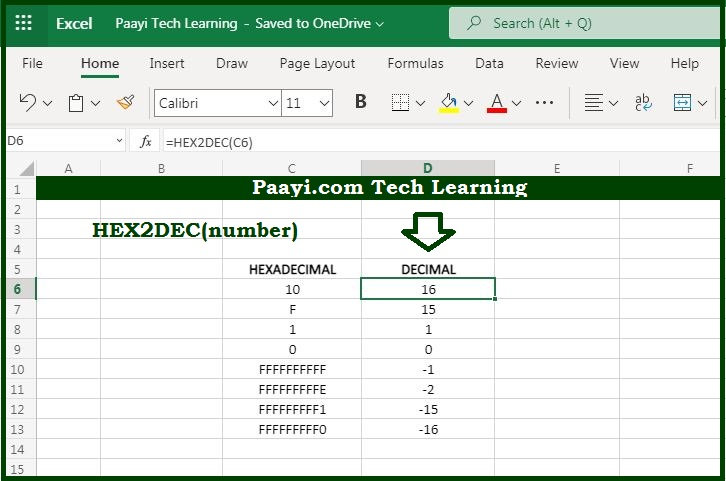# Learn How to Use Microsoft Excel HEX2DEC Function

Written by | 0 Comments | 805 Views

In this article, you will learn how to use the Microsoft Excel HEX2DEC function and its prime function in Microsoft Excel. You will also get to know the Microsoft Excel HEX2DEc function return value and syntax with the help of some examples.

Microsoft Excel HEX2DEC Function

The prime function of the Microsoft Excel HEX2DEC function is to convert hexadecimal numbers to decimal numbers. That implies with the help of the HEX2DEC function you can able to convert the hexadecimal number to its decimal equivalent.

Return Value of HEX2DEC Function

The return value will be the decimal number.

Syntax of HEX2DEC Function

=HEX2DEC(number)

Where the arguments:

number: It is the hexadecimal number that you want to convert into decimal.

## How to Use Microsoft Excel HEX2DEC Function?As we know that HEX2DEC function used to convert hexadecimal numbers to their decimal equivalent. Always keep in mind that:

The input number should be less than or equal to the ten alphanumeric characters, or the function will return the error as #NUM!.

The internal (decimal) representation of the hexadecimal number uses two's complement notation. The first bit shows whether the number is positive or negative, and the rest 39 bits denote the magnitude of the number.